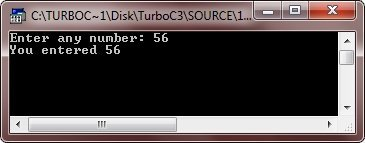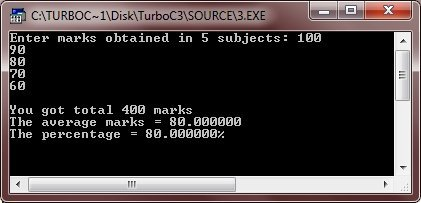# C typedef

In C language, by using the keyword typedef, you can define new data type names. It means, you are not creating a new data type, but rather you are defining a new name for an existing data type. Here is the general form of typedef:

`typedef type new_name;`

Here, typedef is the keyword used in defining new name for an existing data type. And type is any valid data type, new_name is the new name of this data type. Here is an example.

`typedef int num;`

From the above statement, num is the new name for int. This tells the compiler to recognize num as another name for int. Now you can create a int variable using num as shown in this statement:

`num i;`

Here, i is an int variable of type num, which is another word for int.

Now that num has been defined, it can be used in another typedef. For instance, let's look at this statement.

`typedef num over_num;`

tells the compiler to recognize over_num as another name for num, which is another name for int. Now you can again create a int variable using over_num as shown in the following statement:

`over_num a;`

Here, a is an int variable of type over_num, which is another word for num, which is another word for int.

## C typedef Example

Here are some examples listed, demonstrating typedef keyword in C:

```/* C typedef - This program demonstrates the
* use of typedef keyword in creating a new
* name for any existing data type in C
*/

#include<stdio.h>
#include<conio.h>
void main()
{
typedef int num;     // now int has another name i.e., num
num i;
clrscr();

printf("Enter any number: ");
scanf("%d", &i);
printf("You entered %d", i);

getch();
}```

Here is the sample run of the above C program:Here is another example program:

```/* C typedef */

#include<stdio.h>
#include<conio.h>
void main()
{
typedef int num;
typedef num over_num;
over_num a;

clrscr();

printf("Enter a number: ");
scanf("%d", &a);
printf("You entered %d", a);

getch();
}```

Here is the sample run of this C program:Here is one more example program given for complete understanding on typedef keyword.

```#include<stdio.h>
#include<conio.h>
void main()
{
typedef int integer;
typedef float floating_point;
typedef char character;

integer i, sum=0;
integer arr;
floating_point avg;
character ch;

clrscr();

printf("Enter marks obtained in 5 subjects: ");
for(i=0; i<5; i++)
{
scanf("%d", &arr[i]);
sum = sum + arr[i];
}
avg = sum/5;

printf("\nYou got total %d marks", sum);
printf("\nThe average marks = %f", avg);
printf("\nThe percentage = %f%", avg);

getch();
}```

Below is the sample run of this C program.Tools
Calculator